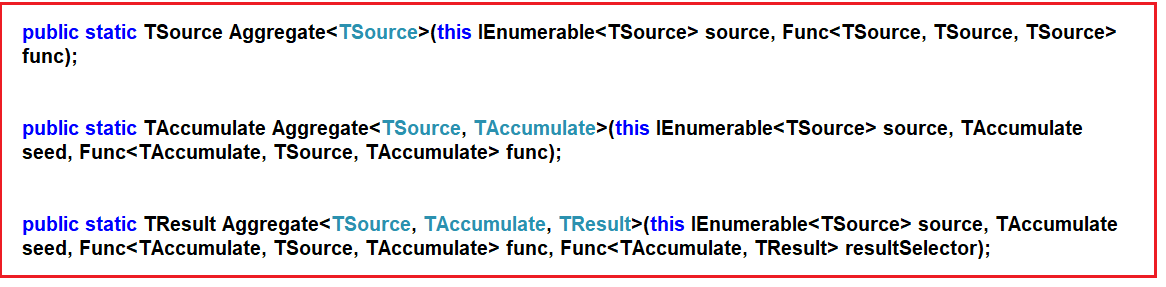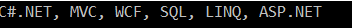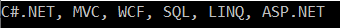# LINQ Aggregate Method in C#

## LINQ Aggregate Method in C# with Examples

In this article, I will discuss the LINQ Aggregate Method in C# with Examples. Please read our previous article before proceeding to this article, where we discussed the LINQ Count Method in C# with Examples. As part of this article, we will discuss the following pointers.

1. What is the LINQ Aggregate Method in C#?
2. Example Comma-Separated String
3. Example Product of Integer Numbers
4. Aggregate Method With the Seed Parameter
5. LINQ Aggregate Method with Complex Type in C#
6. Aggregate Method with Result Selector
7. When to Use LINQ Aggregate Method in C#?
8. When Not to Use LINQ Aggregate Method in C#?
##### What is the LINQ Aggregate Method in C#?

The LINQ Aggregate Method in C# performs a custom aggregation operation on the elements of a sequence. This method applies a function that you define to each element in sequence, carrying the result forward as it processes the elements one by one. It’s essentially a way to “accumulate” a result by applying a function that can combine each item with the current accumulated result.

The LINQ Aggregate extension method performs an accumulative operation. There are three overloaded versions of this method available in the System.Linq namespace, which is shown in the below image.Let us understand the use of the Aggregate method with some examples.

##### Example: Comma-Separated String.

Let us consider we have the following string array
string[] skills = { “C#.NET”, “MVC”, “WCF”, “SQL”, “LINQ”,  “ASP.NET”};

Our requirement is to combine all the above strings in the skill array into a single comma-separated string, as shown below.
C#.NET, MVC, WCF, SQL, LINQ, ASP.NET

###### Program without using the LINQ Aggregate method:
```using System;
using System.Linq;
namespace LINQDemo
{
class Program
{
static void Main(string[] args)
{
string[] skills = { "C#.NET", "MVC", "WCF", "SQL", "LINQ", "ASP.NET" };

string result = string.Empty;

foreach(string skill in skills)
{
result = result + skill + ", ";
}

//Find the index position of last comma
int lastIndex = result.LastIndexOf(",");

//Remove the last comma
result = result.Remove(lastIndex);

Console.WriteLine(result);
}
}
}
```
###### Output:Let us see how to achieve the same output using the Linq Aggregate method.

##### Program using LINQ Aggregate Method in C#:
```using System;
using System.Linq;
namespace LINQDemo
{
class Program
{
static void Main(string[] args)
{
string[] skills = { "C#.NET", "MVC", "WCF", "SQL", "LINQ", "ASP.NET" };

string result = skills.Aggregate((s1, s2) => s1 + ", " + s2);

Console.WriteLine(result);
}
}
}
```
###### Output:##### How Does the Above LINQ Aggregate Method Work?

The lambda expression (s1, s2) => s1 + “, ” + s2 will be treated like s1 = s1 + “, ” + s2, where s1 will be accumulated for each item present in the collection. As a result, the Aggregate function will return the comma-separated string. Please look at how the comma-separated string is generated step by step.

1. Step 1: First, “C#.NET” is concatenated with “MVC” to produce the result “C#.NET, MVC.”
2. Step 2: The result in Step 1, i.e., “C#.NET, MVC,” is then concatenated with “WCF” to produce the result “C#.NET, MVC, WCF.”
3. Step 3: The result in Step 2, i.e., “C#.NET, MVC, WCF,” is then concatenated with “SQL” to produce the result “C#.NET, MVC, WCF, SQL.”
4. Step 4: The result in Step 3, i.e., “C#.NET, MVC, WCF, SQL,” is then concatenated with “LINQ” to produce the result “C#.NET, MVC, WCF, SQL, LINQ.”
5. Step 5: The result in Step 4, i.e., “C#.NET, MVC, WCF, SQL, LINQ,” is then concatenated with “ASP.NET” to produce the final result “C#.NET, MVC, WCF, SQL, LINQ, ASP.NET” what we see in the output.
##### Example: Product of Integer Numbers

Consider we have the following integer array
int[] intNumbers = { 3, 5, 7, 9 };

Our requirement is to compute the product of all numbers.

##### Program Without using LINQ Aggregate Method in C#:
```namespace LINQDemo
{
class Program
{
static void Main(string[] args)
{
int[] intNumbers = { 3, 5, 7, 9 };

int result = 1;
foreach(int num in intNumbers)
{
result = result * num;
}

Console.WriteLine(result);
}
}
}
```
##### Program using LINQ Aggregate Method in C#:
```using System;
using System.Linq;
namespace LINQDemo
{
class Program
{
static void Main(string[] args)
{
int[] intNumbers = { 3, 5, 7, 9 };

int result = intNumbers.Aggregate((n1, n2) => n1 * n2);

Console.WriteLine(result);
}
}
}
```

How does the above Aggregate Method work?

1. Step 1: First, it multiplies (3X5) to produce the result of 15
2. Step 2: The result of Step 1, i.e., 15, is then multiplied by 7 to produce the result as 105
3. Step 3: The result of Step 2, i.e., 105, is multiplied by 9 to produce the final result as 945.
##### Aggregate Method With the Seed Parameter:

The second overloaded version of the Aggregate method takes the first parameter as the seed value to accumulate. The Second parameter is Func type delegate: Let us understand the use of the seed parameter with an example. Let us see how to pass the seed value as 2 with our previous example.

int result = intNumbers.Aggregate(2, (n1, n2) => n1 * n2);

The complete example is given below.

```using System;
using System.Linq;
namespace LINQDemo
{
class Program
{
static void Main(string[] args)
{
int[] intNumbers = { 3, 5, 7, 9 };

int result = intNumbers.Aggregate(2, (n1, n2) => n1 * n2);

Console.WriteLine(result);
}
}
}
```

Output: 1890

##### How Does It Work?
1. Step 1: First, it multiplies (2*3) to produce the result as 6
2. Step 2: The result of Step 1, i.e., 6, is then multiplied by 5 to produce the result as 30
3. Step 3: The result of Step 2, i.e., 30, is then multiplied by 7 to produce the result as 210.
4. Step 4: The result of Step 3, i.e., 210, is then multiplied by 9 to produce the final result as 1890.

The Aggregate method can be considered a general-purpose tool for producing a single, cumulative result from a sequence. It’s a powerful function that can be used for a variety of tasks beyond just summing numbers, including but not limited to:

• Multiplying all elements together to get a product.
• Combining strings or building a custom string representation of a sequence.
• Determining minimum or maximum values under custom rules.
• Accumulating a complex object, like merging a list of objects into a single summary object.
##### LINQ Aggregate Method with Complex Type in C#:

We are going to work with the following Employee class.

```using System.Collections.Generic;
namespace LINQDemo
{
public class Employee
{
public int ID { get; set; }
public string Name { get; set; }
public int Salary { get; set; }
public string Department { get; set; }

public static List<Employee> GetAllEmployees()
{
List<Employee> listStudents = new List<Employee>()
{
new Employee{ID= 101,Name = "Preety", Salary = 10000, Department = "IT"},
new Employee{ID= 102,Name = "Priyanka", Salary = 15000, Department = "Sales"},
new Employee{ID= 103,Name = "James", Salary = 50000, Department = "Sales"},
new Employee{ID= 104,Name = "Hina", Salary = 20000, Department = "IT"},
new Employee{ID= 105,Name = "Anurag", Salary = 30000, Department = "IT"},

};

return listStudents;
}
}
}```

While working with the complex type, we need to use either the second or third overloaded version of the Aggregate method. The following example uses the Aggregate method to add the salary of all the employees.

```using System;
using System.Linq;
namespace LINQDemo
{
class Program
{
static void Main(string[] args)
{
int Salary = Employee.GetAllEmployees()
.Aggregate<Employee, int>(0,
(TotalSalary, emp) => TotalSalary += emp.Salary);

Console.WriteLine(Salary);
}
}
}
```

Please note here, we passed the seed value as 0. Once you run the application, it gives you the output as expected. In the following example, we pass a string as the seed value to the Aggregate extension method. Here, the seed value is “Employee Names”.

```using System;
using System.Linq;
namespace LINQDemo
{
class Program
{
static void Main(string[] args)
{
string CommaSeparatedEmployeeNames = Employee.GetAllEmployees().Aggregate<Employee, string>(
"Employee Names: ",  // seed value
(employeeNames, employee) => employeeNames = employeeNames + employee.Name + ", ");

int LastIndex = CommaSeparatedEmployeeNames.LastIndexOf(",");
CommaSeparatedEmployeeNames = CommaSeparatedEmployeeNames.Remove(LastIndex);

Console.WriteLine(CommaSeparatedEmployeeNames);
}
}
}
```

Output: Employee Names: Preety, Priyanka, James, Hina, Anurag

In the above example, the first parameter of the Aggregate method is the “Employee Names: ” string that will be accumulated with all employee names. The comma in the lambda expression will be passed as the second parameter.

##### Aggregate Method with Result Selector

The third overload version requires the third parameter of the Func delegate expression for the result selector to formulate the result. In our previous example, once we get the comma-separated string, we remove the last comma using some additional logic. Let us see how we can do the same using the third parameter.

```using System;
using System.Linq;
namespace LINQDemo
{
class Program
{
static void Main(string[] args)
{
string CommaSeparatedEmployeeNames = Employee.GetAllEmployees().Aggregate<Employee, string, string>(
"Employee Names: ",  // seed value
(employeeNames, employee) => employeeNames = employeeNames + employee.Name + ", ",
employeeNames => employeeNames.Substring(0, employeeNames.Length - 1));

Console.WriteLine(CommaSeparatedEmployeeNames);
}
}
}
```

Output: Employee Names: Preety, Priyanka, James, Hina, Anurag

In the above example, we have specified a lambda expression, i.e., employeeNames => employeeNames.Substring(0, employeeNames.Length – 1) to remove the last comma in the string result.

##### When to Use LINQ Aggregate Method in C#?

Here are some situations where Aggregate is particularly appropriate:

• Cumulative Operations: When you need to apply an operation to each item in a collection, accumulating a result, such as finding the product of all numbers in a list.
• String Concatenation: Building a single string from a collection of strings, such as creating a CSV from a list of values.
• Custom Aggregations: When built-in aggregate functions like Sum, Min, Max, or Average are not sufficient, and you need a more complex or specific aggregation logic.
• Data Transformation: To transform a collection into a single, more complex object, like combining a list of key-value pairs into a dictionary.
• Running Totals: To compute running totals or other progressive calculations that require the result of the previous step to calculate the next step.
• Hierarchical Data: When you have a tree or graph structure, you want to aggregate information across nodes, such as summing values across a tree.
• Sequences with a Single Element: If you need to perform an action on a sequence expected to have a single item, Aggregate can apply a function and return that item directly.

In the next article, I will discuss the Quantifier Operators in LINQ with examples. In this article, I explain the LINQ Aggregate Method in C# with Examples, and I hope you understand the need and use of the LINQ Aggregate Method in C#.

### 3 thoughts on “LINQ Aggregate Method in C#”

1.Great article. You have covered all the aspect of Aggregate function.

2.Hi. In chapter “Aggregate Method with the seed parameter:” you have a misprint.
You write: “Output: 1980” but really: “Output: 1890”

1.Hi
Thanks for Identifying the Type Error. We have corrected the same.